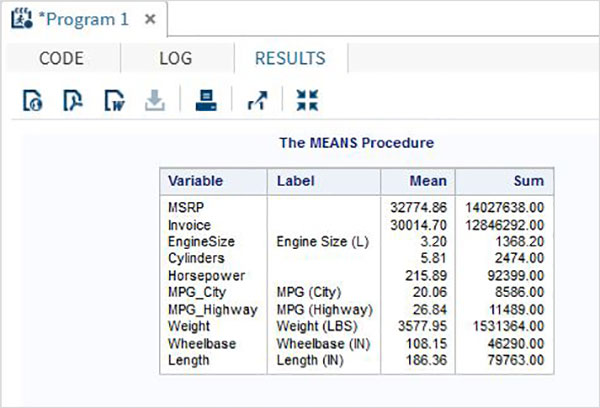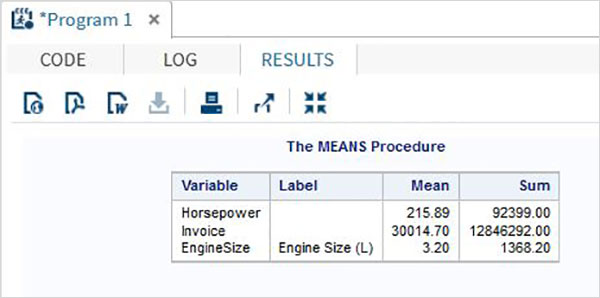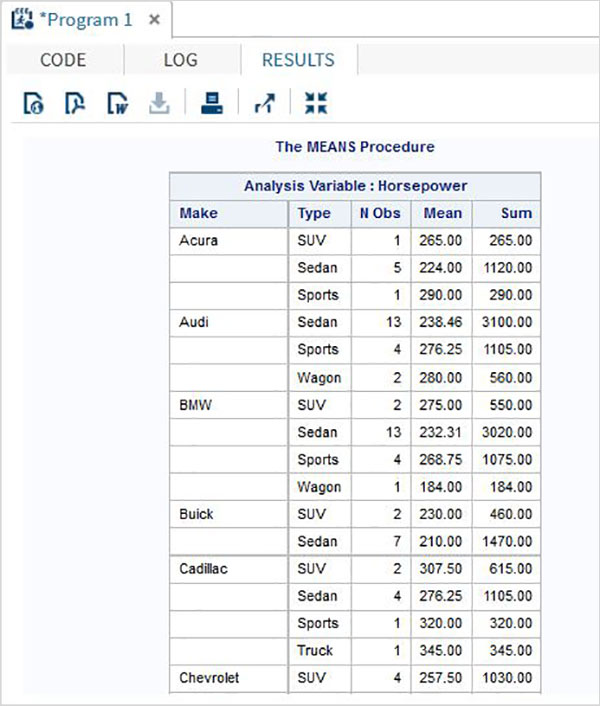# SAS - Arithmetic Mean

The arithmetic mean is the value obtained by summing value of numeric variables and then dividing the sum with the number of variables. It is also called Average. In SAS arithmetic mean is calculated using PROC MEANS. Using this SAS procedure we can find the mean of all variables or some variables of a dataset. We can also form groups and find mean of variables of values specific to that group.

## Syntax

The basic syntax for calculating arithmetic mean in SAS is −

```PROC MEANS DATA = DATASET;
CLASS Variables ;
VAR Variables;
```

Following is the description of parameters used −

• DATASET − is the name of the dataset used.

• Variables − are the name of the variable from the dataset.

## Mean of a Dataset

The mean of each of the numeric variable in a dataset is calculated by using the PROC by supplying only the dataset name without any variables.

### Example

In the below example we find the mean of all the numeric variables in the SAS dataset named CARS. We specify the maximum digits after decimal place to be 2 and also find the sum of those variables.

```PROC MEANS DATA = sashelp.CARS Mean SUM MAXDEC=2;
RUN;
```

When the above code is executed, we get the following output −## Mean of Select Variables

We can get the mean of some of the variables by supplying their names in the var option.

## Example

In the below we calculate the mean of three variables.

```PROC MEANS DATA = sashelp.CARS mean SUM MAXDEC=2 ;
var horsepower invoice EngineSize;
RUN;
```

When the above code is executed, we get the following output −## Mean by Class

We can find the mean of the numeric variables by organizing them to groups by using some other variables.

### Example

In the example below we find the mean of the variable horsepower for each type under each make of the car.

```PROC MEANS DATA = sashelp.CARS mean SUM MAXDEC=2;
class make type;
var horsepower;
RUN;
```

When the above code is executed, we get the following output −